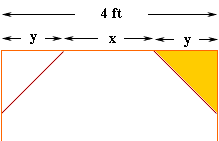Quandaries and Queries I'm doing a stain glass project and it's on a 4 foot across octagonal window...and I'm trying to set up the pattern and I don't know how long the sides are! Could you help me please? Thanks... Mom who's forgotten everything! Kay Hi Kay, And I'll bet you never thought you would need Pythagoras' theorem again! It is amazing how this theorem pops up in unexpected places. I drew a picture of the top section of your window. In the diagram x is the length of each side.Think of forming the octagon by cutting off triangular corners from a square, the yellow triangle above. Let y be the side length of the triangle. From the diagram I see that 4 = y + x + y = x + 2y The triangle you cut off is a right triangle with side length y and y and a hypotenuse of length x. Thus, by Pythagoras' theorem y2 + y2 = x2, that is 2y2 = x2 or y = x/Sqrt(2) where Sqrt(2) is the square root of 2. Substitution into the first equation and a little simplification gives x = 4/1 + Sqrt(2) A calculator then gives x = 1.657 feet. This is approximately 1 foot, 7 and 7/8 inches. Thus if you make the sides 1 foot, 7 and 7/8 inches you should have the octagon you want. Now I want something from you. When you complete the window, send us a picture. We often get, from students, the question "when will I ever use Pythagoras' theorem in real life?". Your question is a nice answer but would be even better with an image of the window. Penny Go to Math Central